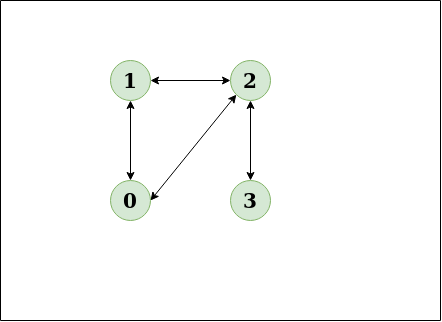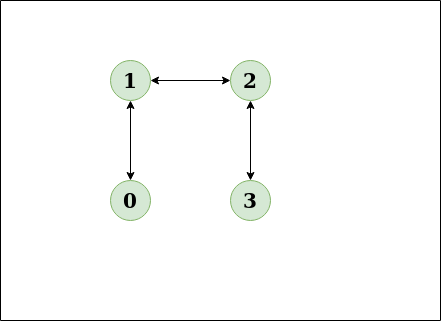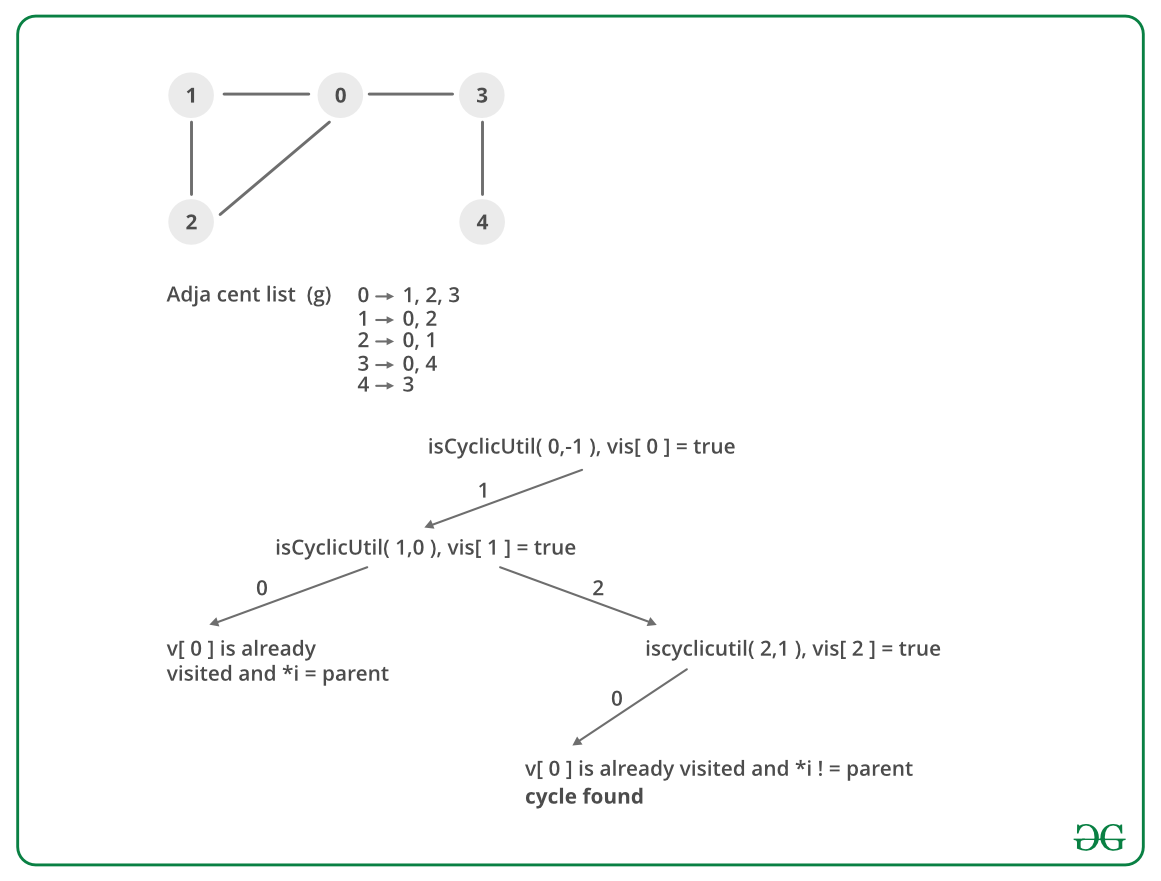Related Articles

# Detect cycle in an undirected graph

• Difficulty Level : Medium
• Last Updated : 01 Jul, 2021

Given an undirected graph, how to check if there is a cycle in the graph?
Example,

Input: n = 4, e = 4
Output: Yes
Explanation:
0 1, 1 2, 2 3, 0 2
Diagram:The diagram clearly shows a cycle 0 to 2 to 1 to 0
Input:n = 4, e = 3
0 1, 1 2, 2 3
Output:No
Explanation:
Diagram:The diagram clearly shows no cycle

Approach: Run a DFS from every unvisited node. Depth First Traversal can be used to detect a cycle in a Graph. DFS for a connected graph produces a tree. There is a cycle in a graph only if there is a back edge present in the graph. A back edge is an edge that is joining a node to itself (self-loop) or one of its ancestor in the tree produced by DFS.
To find the back edge to any of its ancestor keep a visited array and if there is a back edge to any visited node then there is a loop and return true.
Algorithm:

1. Create the graph using the given number of edges and vertices.
2. Create a recursive function that have current index or vertex, visited array and parent node.
3. Mark the current node as visited .
4. Find all the vertices which are not visited and are adjacent to the current node. Recursively call the function for those vertices, If the recursive function returns true return true.
5. If the adjacent node is not parent and already visited then return true.
6. Create a wrapper class, that calls the recursive function for all the vertices and if any function returns true, return true.
7. Else if for all vertices the function returns false return false.

Dry Run:Implementation:

## C++

 `// A C++ Program to detect``// cycle in an undirected graph``#include``#include ``#include ``using` `namespace` `std;` `// Class for an undirected graph``class` `Graph``{``    ` `    ``// No. of vertices``    ``int` `V;  ``  ` `    ``// Pointer to an array``    ``// containing adjacency lists``    ``list<``int``> *adj; ``    ``bool` `isCyclicUtil(``int` `v, ``bool` `visited[],``                              ``int` `parent);``public``:``  ` `    ``// Constructor``    ``Graph(``int` `V);  ``  ` `    ``// To add an edge to graph``    ``void` `addEdge(``int` `v, ``int` `w);``  ` `    ``// Returns true if there is a cycle``    ``bool` `isCyclic(); ``};` `Graph::Graph(``int` `V)``{``    ``this``->V = V;``    ``adj = ``new` `list<``int``>[V];``}` `void` `Graph::addEdge(``int` `v, ``int` `w)``{``    ` `    ``// Add w to v’s list.``    ``adj[v].push_back(w);``  ` `    ``// Add v to w’s list.``    ``adj[w].push_back(v);``}` `// A recursive function that``// uses visited[] and parent to detect``// cycle in subgraph reachable``// from vertex v.``bool` `Graph::isCyclicUtil(``int` `v,``                ``bool` `visited[], ``int` `parent)``{``    ` `    ``// Mark the current node as visited``    ``visited[v] = ``true``;` `    ``// Recur for all the vertices``    ``// adjacent to this vertex``    ``list<``int``>::iterator i;``    ``for` `(i = adj[v].begin(); i !=``                       ``adj[v].end(); ++i)``    ``{``        ` `        ``// If an adjacent vertex is not visited,``        ``//then recur for that adjacent``        ``if` `(!visited[*i])``        ``{``           ``if` `(isCyclicUtil(*i, visited, v))``              ``return` `true``;``        ``}` `        ``// If an adjacent vertex is visited and``        ``// is not parent of current vertex,``        ``// then there exists a cycle in the graph.``        ``else` `if` `(*i != parent)``           ``return` `true``;``    ``}``    ``return` `false``;``}` `// Returns true if the graph contains``// a cycle, else false.``bool` `Graph::isCyclic()``{``    ` `    ``// Mark all the vertices as not``    ``// visited and not part of recursion``    ``// stack``    ``bool` `*visited = ``new` `bool``[V];``    ``for` `(``int` `i = 0; i < V; i++)``        ``visited[i] = ``false``;` `    ``// Call the recursive helper``    ``// function to detect cycle in different``    ``// DFS trees``    ``for` `(``int` `u = 0; u < V; u++)``    ``{``      ` `        ``// Don't recur for u if``        ``// it is already visited``        ``if` `(!visited[u])``          ``if` `(isCyclicUtil(u, visited, -1))``             ``return` `true``;``    ``}``    ``return` `false``;``}` `// Driver program to test above functions``int` `main()``{``    ``Graph g1(5);``    ``g1.addEdge(1, 0);``    ``g1.addEdge(0, 2);``    ``g1.addEdge(2, 1);``    ``g1.addEdge(0, 3);``    ``g1.addEdge(3, 4);``    ``g1.isCyclic()?``       ``cout << ``"Graph contains cycle\n"``:``       ``cout << ``"Graph doesn't contain cycle\n"``;` `    ``Graph g2(3);``    ``g2.addEdge(0, 1);``    ``g2.addEdge(1, 2);``    ``g2.isCyclic()?``       ``cout << ``"Graph contains cycle\n"``:``       ``cout << ``"Graph doesn't contain cycle\n"``;` `    ``return` `0;``}`

## Java

 `// A Java Program to detect cycle in an undirected graph``import` `java.io.*;``import` `java.util.*;``// This class represents a``// directed graph using adjacency list``// representation``class` `Graph``{``  ` `    ``// No. of vertices``    ``private` `int` `V;  ``  ` `    ``// Adjacency List Represntation``    ``private` `LinkedList adj[];` `    ``// Constructor``    ``Graph(``int` `v)``    ``{``        ``V = v;``        ``adj = ``new` `LinkedList[v];``        ``for``(``int` `i=``0``; i it =``                ``adj[v].iterator();``        ``while` `(it.hasNext())``        ``{``            ``i = it.next();` `            ``// If an adjacent is not``            ``// visited, then recur for that``            ``// adjacent``            ``if` `(!visited[i])``            ``{``                ``if` `(isCyclicUtil(i, visited, v))``                    ``return` `true``;``            ``}` `            ``// If an adjacent is visited``            ``// and not parent of current``            ``// vertex, then there is a cycle.``            ``else` `if` `(i != parent)``                ``return` `true``;``        ``}``        ``return` `false``;``    ``}` `    ``// Returns true if the graph``    ``// contains a cycle, else false.``    ``Boolean isCyclic()``    ``{``        ` `        ``// Mark all the vertices as``        ``// not visited and not part of``        ``// recursion stack``        ``Boolean visited[] = ``new` `Boolean[V];``        ``for` `(``int` `i = ``0``; i < V; i++)``            ``visited[i] = ``false``;` `        ``// Call the recursive helper``        ``// function to detect cycle in``        ``// different DFS trees``        ``for` `(``int` `u = ``0``; u < V; u++)``        ``{ ``        ` `            ``// Don't recur for u if already visited``            ``if` `(!visited[u])``                ``if` `(isCyclicUtil(u, visited, -``1``))``                    ``return` `true``;``        ``}` `        ``return` `false``;``    ``}`  `    ``// Driver method to test above methods``    ``public` `static` `void` `main(String args[])``    ``{``        ` `        ``// Create a graph given``        ``// in the above diagram``        ``Graph g1 = ``new` `Graph(``5``);``        ``g1.addEdge(``1``, ``0``);``        ``g1.addEdge(``0``, ``2``);``        ``g1.addEdge(``2``, ``1``);``        ``g1.addEdge(``0``, ``3``);``        ``g1.addEdge(``3``, ``4``);``        ``if` `(g1.isCyclic())``            ``System.out.println("Graph``                             ``contains cycle");``        ``else``            ``System.out.println("Graph``                        ``doesn't contains cycle");` `        ``Graph g2 = ``new` `Graph(``3``);``        ``g2.addEdge(``0``, ``1``);``        ``g2.addEdge(``1``, ``2``);``        ``if` `(g2.isCyclic())``            ``System.out.println("Graph``                             ``contains cycle");``        ``else``            ``System.out.println("Graph``                        ``doesn't contains cycle");``    ``}``}``// This code is contributed by Aakash Hasija`

## Python

 `# Python Program to detect cycle in an undirected graph``from` `collections ``import` `defaultdict`` ` `# This class represents a undirected``# graph using adjacency list representation``class` `Graph:`` ` `    ``def` `__init__(``self``,vertices):``    ` `        ``# No. of vertices``        ``self``.V``=` `vertices ``#No. of vertices``        ` `        ``# Default dictionary to store graph``        ``self``.graph ``=` `defaultdict(``list``)` ` ` `    ``# Function to add an edge to graph``    ``def` `addEdge(``self``,v,w):``      ` `        ``#Add w to v_s list``        ``self``.graph[v].append(w)``        ` `         ``#Add v to w_s list``        ``self``.graph[w].append(v)`` ` `    ``# A recursive function that uses``    ``# visited[] and parent to detect``    ``# cycle in subgraph reachable from vertex v.``    ``def` `isCyclicUtil(``self``,v,visited,parent):` `        ``# Mark the current node as visited``        ``visited[v]``=` `True` `        ``# Recur for all the vertices``        ``# adjacent to this vertex``        ``for` `i ``in` `self``.graph[v]:``            ` `            ``# If the node is not``            ``# visited then recurse on it``            ``if`  `visited[i]``=``=``False` `:``                ``if``(``self``.isCyclicUtil(i,visited,v)):``                    ``return` `True``            ``# If an adjacent vertex is``            ``# visited and not parent``            ``# of current vertex,``            ``# then there is a cycle``            ``elif`  `parent!``=``i:``                ``return` `True``        ` `        ``return` `False``         ` ` ` `    ``# Returns true if the graph``    ``# contains a cycle, else false.``    ``def` `isCyclic(``self``):``      ` `        ``# Mark all the vertices``        ``# as not visited``        ``visited ``=``[``False``]``*``(``self``.V)``        ` `        ``# Call the recursive helper``        ``# function to detect cycle in different``        ``# DFS trees``        ``for` `i ``in` `range``(``self``.V):``          ` `            ``# Don't recur for u if it``            ``# is already visited``            ``if` `visited[i] ``=``=``False``:``                ``if``(``self``.isCyclicUtil``                       ``(i,visited,``-``1``)) ``=``=` `True``:``                    ``return` `True``        ` `        ``return` `False` `# Create a graph given in the above diagram``g ``=` `Graph(``5``)``g.addEdge(``1``, ``0``)``g.addEdge(``1``, ``2``)``g.addEdge(``2``, ``0``)``g.addEdge(``0``, ``3``)``g.addEdge(``3``, ``4``)` `if` `g.isCyclic():``    ``print` `"Graph contains cycle"``else` `:``    ``print` `"Graph does not contain cycle "``g1 ``=` `Graph(``3``)``g1.addEdge(``0``,``1``)``g1.addEdge(``1``,``2``)`  `if` `g1.isCyclic():``    ``print` `"Graph contains cycle"``else` `:``    ``print` `"Graph does not contain cycle "`` ` `#This code is contributed by Neelam Yadav`

## C#

 `// C# Program to detect cycle in an undirected graph``using` `System;``using` `System.Collections.Generic;` `// This class represents a directed graph``// using adjacency list representation``class` `Graph``{``    ``private` `int` `V; ``// No. of vertices``    ` `    ``// Adjacency List Represntation``    ``private` `List<``int``> []adj;` `    ``// Constructor``    ``Graph(``int` `v)``    ``{``        ``V = v;``        ``adj = ``new` `List<``int``>[v];``        ``for``(``int` `i = 0; i < v; ++i)``            ``adj[i] = ``new` `List<``int``>();``    ``}` `    ``// Function to add an edge into the graph``    ``void` `addEdge(``int` `v, ``int` `w)``    ``{``        ``adj[v].Add(w);``        ``adj[w].Add(v);``    ``}` `    ``// A recursive function that uses visited[]``    ``// and parent to detect cycle in subgraph``    ``// reachable from vertex v.``    ``Boolean isCyclicUtil(``int` `v, Boolean []visited,``                         ``int` `parent)``    ``{``        ``// Mark the current node as visited``        ``visited[v] = ``true``;` `        ``// Recur for all the vertices``        ``// adjacent to this vertex``        ``foreach``(``int` `i ``in` `adj[v])``        ``{``            ``// If an adjacent is not visited,``            ``// then recur for that adjacent``            ``if` `(!visited[i])``            ``{``                ``if` `(isCyclicUtil(i, visited, v))``                    ``return` `true``;``            ``}` `            ``// If an adjacent is visited and``            ``// not parent of current vertex,``            ``// then there is a cycle.``            ``else` `if` `(i != parent)``                ``return` `true``;``        ``}``        ``return` `false``;``    ``}` `    ``// Returns true if the graph contains``    ``// a cycle, else false.``    ``Boolean isCyclic()``    ``{``        ``// Mark all the vertices as not visited``        ``// and not part of recursion stack``        ``Boolean []visited = ``new` `Boolean[V];``        ``for` `(``int` `i = 0; i < V; i++)``            ``visited[i] = ``false``;` `        ``// Call the recursive helper function``        ``// to detect cycle in different DFS trees``        ``for` `(``int` `u = 0; u < V; u++)``        ` `            ``// Don't recur for u if already visited``            ``if` `(!visited[u])``                ``if` `(isCyclicUtil(u, visited, -1))``                    ``return` `true``;` `        ``return` `false``;``    ``}` `    ``// Driver Code``    ``public` `static` `void` `Main(String []args)``    ``{``        ``// Create a graph given in the above diagram``        ``Graph g1 = ``new` `Graph(5);``        ``g1.addEdge(1, 0);``        ``g1.addEdge(0, 2);``        ``g1.addEdge(2, 1);``        ``g1.addEdge(0, 3);``        ``g1.addEdge(3, 4);``        ``if` `(g1.isCyclic())``            ``Console.WriteLine(``"Graph contains cycle"``);``        ``else``            ``Console.WriteLine(``"Graph doesn't contains cycle"``);` `        ``Graph g2 = ``new` `Graph(3);``        ``g2.addEdge(0, 1);``        ``g2.addEdge(1, 2);``        ``if` `(g2.isCyclic())``            ``Console.WriteLine(``"Graph contains cycle"``);``        ``else``            ``Console.WriteLine(``"Graph doesn't contains cycle"``);``    ``}``}` `// This code is contributed by PrinciRaj1992`

Output:

```Graph contains cycle
Graph doesn't contain cycle```

Complexity Analysis:

• Time Complexity: O(V+E).
The program does a simple DFS Traversal of the graph which is represented using adjacency list. So the time complexity is O(V+E).
• Space Complexity: O(V).
To store the visited array O(V) space is required.

Exercise: Can we use BFS to detect cycle in an undirected graph in O(V+E) time? What about directed graphs?